Courses

# Moment of Inertia and area about Inclined Axis Mechanical Engineering Notes | EduRev

## Mechanical Engineering : Moment of Inertia and area about Inclined Axis Mechanical Engineering Notes | EduRev

The document Moment of Inertia and area about Inclined Axis Mechanical Engineering Notes | EduRev is a part of the Mechanical Engineering Course Engineering Mechanics - Notes, Videos, MCQs & PPTs.
All you need of Mechanical Engineering at this link: Mechanical Engineering

Moments of Inertia about inclined axis

In structural and mechanical design, it is sometimes necessary to calculate the moment of inertia with respect to a set of inclined u, v, axes when the values of q , Ix, Iy, Ixy are known.

To do this we will use transformation equations which relates the x, y, and x’, y’ coordinates.

From Figure, these equations are:

Note: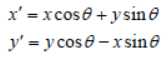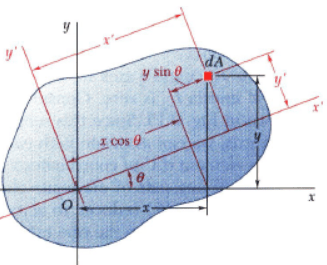Moments of Inertia about inclined axis,, continue

Given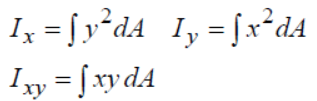we wish to determine moments and product of inertia with respect to new axes x’ and y’.

• The change of axes yields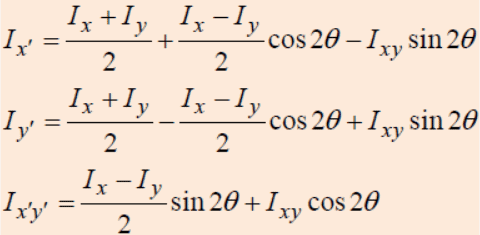• By adding the equations for Ix’ and Ix’ we can show that the polar moment of inertia about z axis passing through point O is independent of the orientation of x’ and y;

 Jo = Ix’ + Iy’ = Ix + Iy

• These equations show that Ix’ , Iy’ and Ix’y’ depend on the angle of the inclination, θ, of the x’, y’ axes.

Principal Axes and Principal Moments of Inertia

We will now determine the orientation of these axes about which Ix’ , Iy’ are maximum and minimum. This particular axes are called principal axes By differentiating the first of Eqs. 10-9 with respect to q and setting the result to zero. Thus;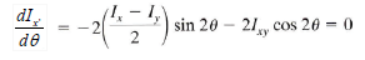Therefore, at θ = θp ;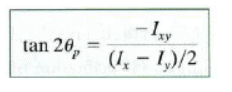By substituting for θ in Ix’ , Iy’ and Ix’y’ equations and simplifying, we obtain;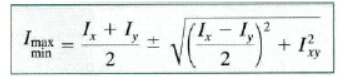Summary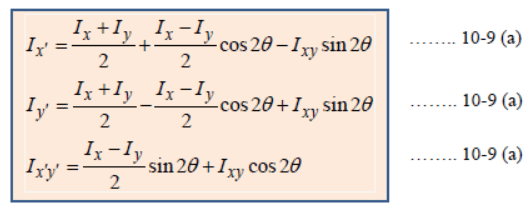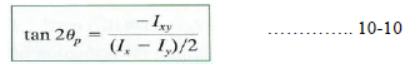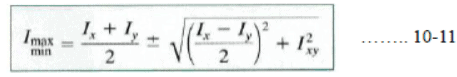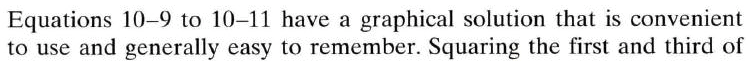Offer running on EduRev: Apply code STAYHOME200 to get INR 200 off on our premium plan EduRev Infinity!

,

,

,

,

,

,

,

,

,

,

,

,

,

,

,

,

,

,

,

,

,

;